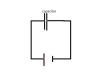# Capacitor - Work done to charge it up

• aaaa202
In summary, the capacitor stores energy by taking a tiny chunk of charge and separating it from the one plate to the other. The work done to charge the capacitor is proportional to the potential difference between the plates.f

#### aaaa202

capacitor -- Work done to charge it up

If we imagine a capacitor as two conductors held a distance d apart from each other, we can find easily that the total charge and the potential are proportional:
Q = CV
To find the energy stored in a capacitor you can imagine taking a tiny chunk of charge and separating it from the one plate to the other. The work you must do is:
Q/Cdq and you can integrate up to find the total work needed done.

To me however, there is some subleties in this definition of the energy stored in it:
Because, doesn't it matter from where on the conductor you take your negative charges and transport them to the positive one? Imagine we have a capacitor made of some conductors of weird shape. Generally the field between them can vary depending not only on the distance between them but also on where you are on the conductors. So the work needed for taking an electron from one and transporting to the other will vary depending on which electron you pick. What reference point on the capacitor should be used?

Each conductor has the same potential everywhere on its surface or inside it, assuming that we transfer charge slowly enough that the setup stays infinitesmally close to an electrostatic situation.

hmm yes, so you get like a quasistatic situation. But I don't think it's very obvious though. So say we have a simple circuit like the below. The platecapacitor is connected to the battery with wires, which are of course also conductors. Since the wire and plate is an equipotential does that then mean that the line integral of the electric field from either of the red dot to the green dot is the same?

#### Attachments

•capacitor.png
802 bytes · Views: 376
Last edited:

Yes. Remember, there is an electric field inside the battery which is maintained by electrochemical processes.

Yeh okay, I understand what you are saying. The problem is for me that it is kind of a contradicting to say that the work done on the charges in moving inside the conducting part of the circuit is zero since the field inside it is zero - because the charges are moving exactly when the field is not zero as to yet again establish a field equal to zero. And shouldn't this motion give rise to some extra energy, which is not included in the model where you say that the only place, where there is an actual field is inside the battery.